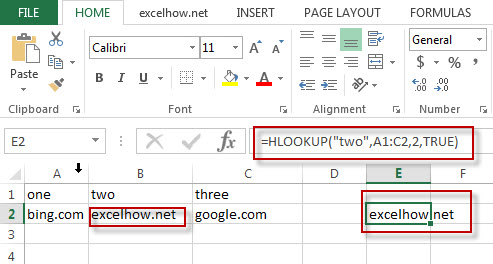Excel Hlookup Function

This post will guide you how to use Excel HLOOKUP function with syntax and examples in Microsoft excel.

Description

The Excel HLOOKUP function lookup a value in the top row of the table and return the value in the same column based on index_num position.

The HLOOKUP function is a build-in function in Microsoft Excel and it is categorized as a Lookup and Reference Function.

The HLOOKUP function is available in Excel 2016, Excel 2013, Excel 2010, Excel 2007, Excel 2003, Excel XP, Excel 2000, Excel 2011 for Mac.

Syntax

The syntax of the HLOOKUP function is as below:

= HLOOKUP (lookup_value, table_array, row_index_num,[range_lookup])

Where the HLOOKUP function arguments are:
Lookup_value -This is a required argument. The value that you want to search for, in the first row of the table. Note: the lookup_value can be a value, a cell or ranges reference, or a text string.
Table_array – This is a required argument. Two or more rows of data to be searched in the top row.
Row_index_num – This is a required argument.  The row number in table_array.
Range_lookup – This is a optional argument.  The value can be set as True or False. If true, the function will return an approximate match value. If False, it will find an exact match or it will return an error value #N/A.

Example

The below examples will show you how to use Excel HLOOKUP Lookup and Reference Function.

#1 To search “two” text string in row 1 and returns the value from row 2 that is in the same column, just using the following excel formula: =HLOOKUP(“two”,A1:C2,2,TRUE)Related Posts

Excel Rows Function

This post will guide you how to use Excel ROWS function with syntax and examples in Microsoft excel. Description The Excel ROWS function returns the number of rows in a cell reference. The ROWS function is a build-in function in Microsoft ...

Excel Row Function

This post will guide you how to use Excel ROW function with syntax and examples in Microsoft excel. Description The Excel ROW function returns the row number of a cell reference. The ROW function is a build-in function in Microsoft ...

Excel Match Function

This post will guide you how to use Excel MATCH function with syntax and examples in Microsoft excel. Description The Excel MATCH function search a value in an array and returns the position of that item. The MATCH function is a ...

Excel Lookup Function

This post will guide you how to use Excel LOOKUP function with syntax and examples in Microsoft excel. Description The Excel LOOKUP function will search a value in a vector or array. The LOOKUP function is a build-in function in Microsoft ...

Excel Indirect Function

This post will guide you how to use Excel INDIRECT function with syntax and examples in Microsoft excel. Description The Excel INDIRECT function returns the cell reference based on a text string, such as: type the text string “A2” in ...

Excel Index Function

This post will guide you how to use Excel INDEX function with syntax and examples in Microsoft excel. Description The Excel INDEX function returns a value from a table based on the index (row number and column number). You can ...

This post will guide you how to use Excel HYPERLINK function with syntax and examples in Microsoft excel. Description The Excel HYPERLINK function creates a shortcut/hyperlink to a document, when you click this hyperlink, the excel will open the file that is stored on ...

Excel Columns Function

This post will guide you how to use Excel COLUMNS function with syntax and examples in Microsoft excel. Description The Excel COLUMNS function returns the number of columns in an Array or a reference. The COLUMNS function is a build-in function ...

Excel Column Function

This post will guide you how to use Excel COLUMN function with syntax and examples in Microsoft excel. Description The Excel COLUMN function returns the first column number of the given cell reference. The COLUMN function is a build-in function in ...

Excel Choose Function

This post will guide you how to use Excel CHOOSE function with syntax and examples in Microsoft excel. Description The Excel CHOOSE function returns a value from a list of values. The CHOOSE function is a build-in function in Microsoft Excel ...

Sidebar